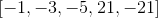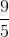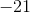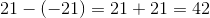# ISEE Upper Level Math : How to find range

## Example Questions

1 3 Next →

### Example Question #21 : How To Find Range

Determine the range:Explanation:

The range is the difference between the largest and smallest numbers.

The largest number is:The smallest number is:Subtract both quantities.The answer is:1 3 Next →